Next: Event-learning with a background Up: -MDPs: Learning in Varying Previous: Sampling from Near-identical Distributions

# Stochastic Processes with Non-diminishing Perturbations

In this appendix we prove the lemma required by the proof of Theorem 3.3:

Lemma C.1   Let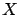be an arbitrary set,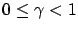,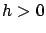and consider the sequence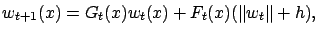where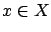, there exists a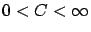bound such that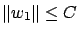with probability one,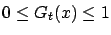and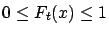for all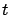. Assume that for all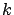,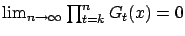uniformly inw.p.1 and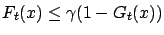w.p.1. Then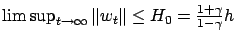w.p.1.

Proof. We will prove that for each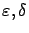there exists an index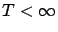such that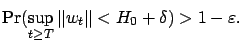(15)

Fix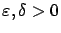arbitrarily. Furthermore fix a sequence of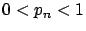numbers (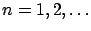) to be chosen later.

Let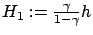and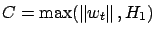. Then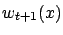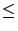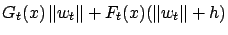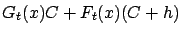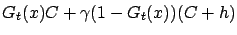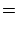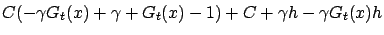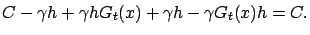Thus, we have that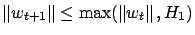. Now define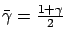. Since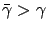,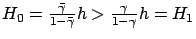. Consequently, if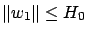, then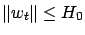holds for all, as well. From now on, we will assume that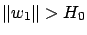.

Let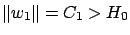. Since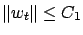, the process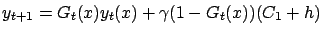with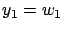estimates the process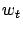from above: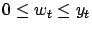holds for all. The process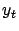converges to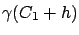w.p.1 uniformly over, so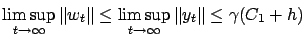w.p.1. Since, there exists an index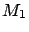, for which if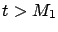then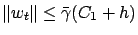with probability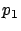. The proof goes on by induction: assume that up to some index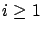we have found indices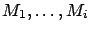such that when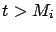then(16)

holds with probability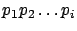. Now let us restrict ourselves to those events for which inequality (16) holds. Then we see that the process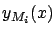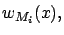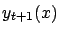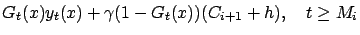boundsfrom above from the index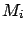. The processconverges to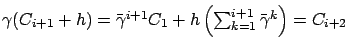w.p.1 uniformly over, so the above argument can be repeated to obtain an index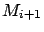such that (16) holds for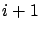with probability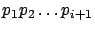.

Since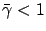,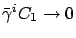and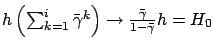. So there exists an indexfor which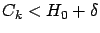. Then inequality (15) can be satisfied by setting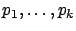so that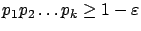holds and letting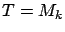.Next: Event-learning with a background Up: -MDPs: Learning in Varying Previous: Sampling from Near-identical Distributions